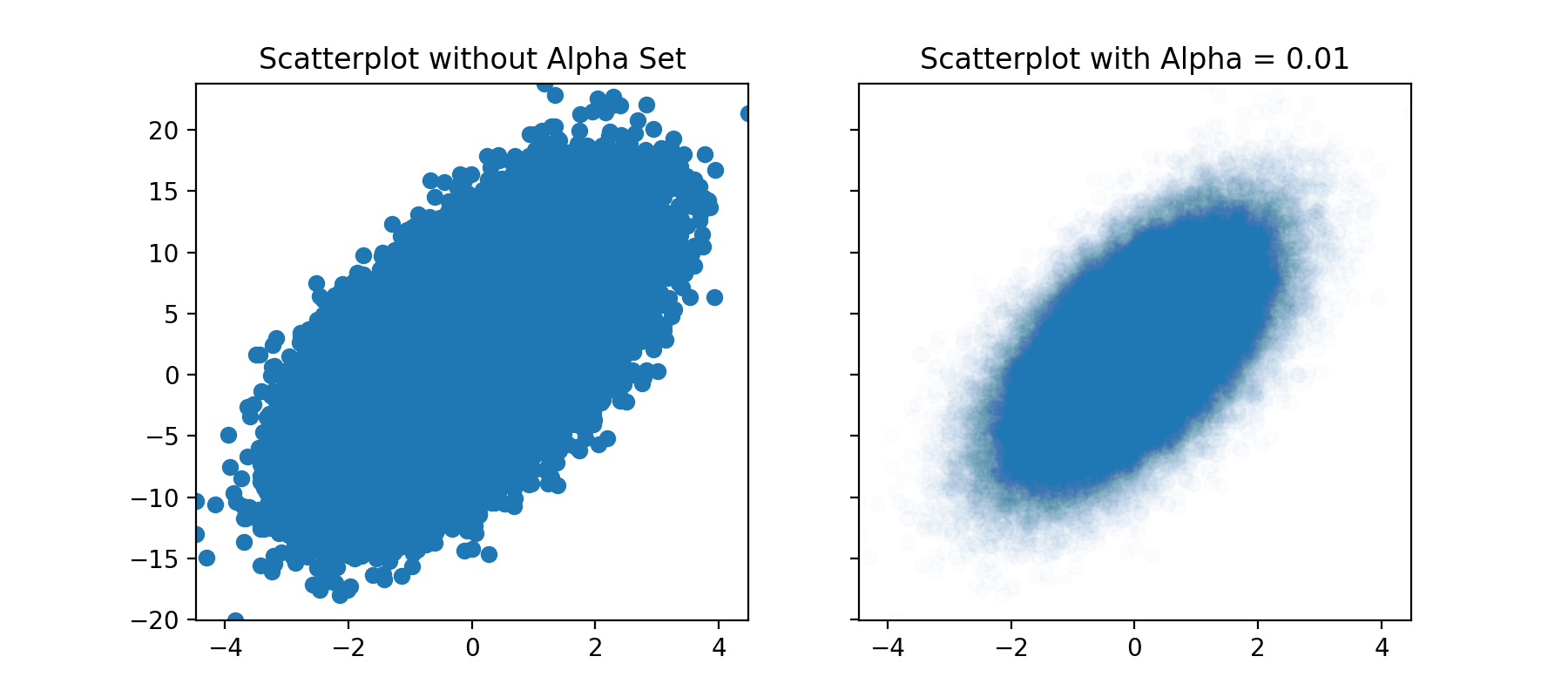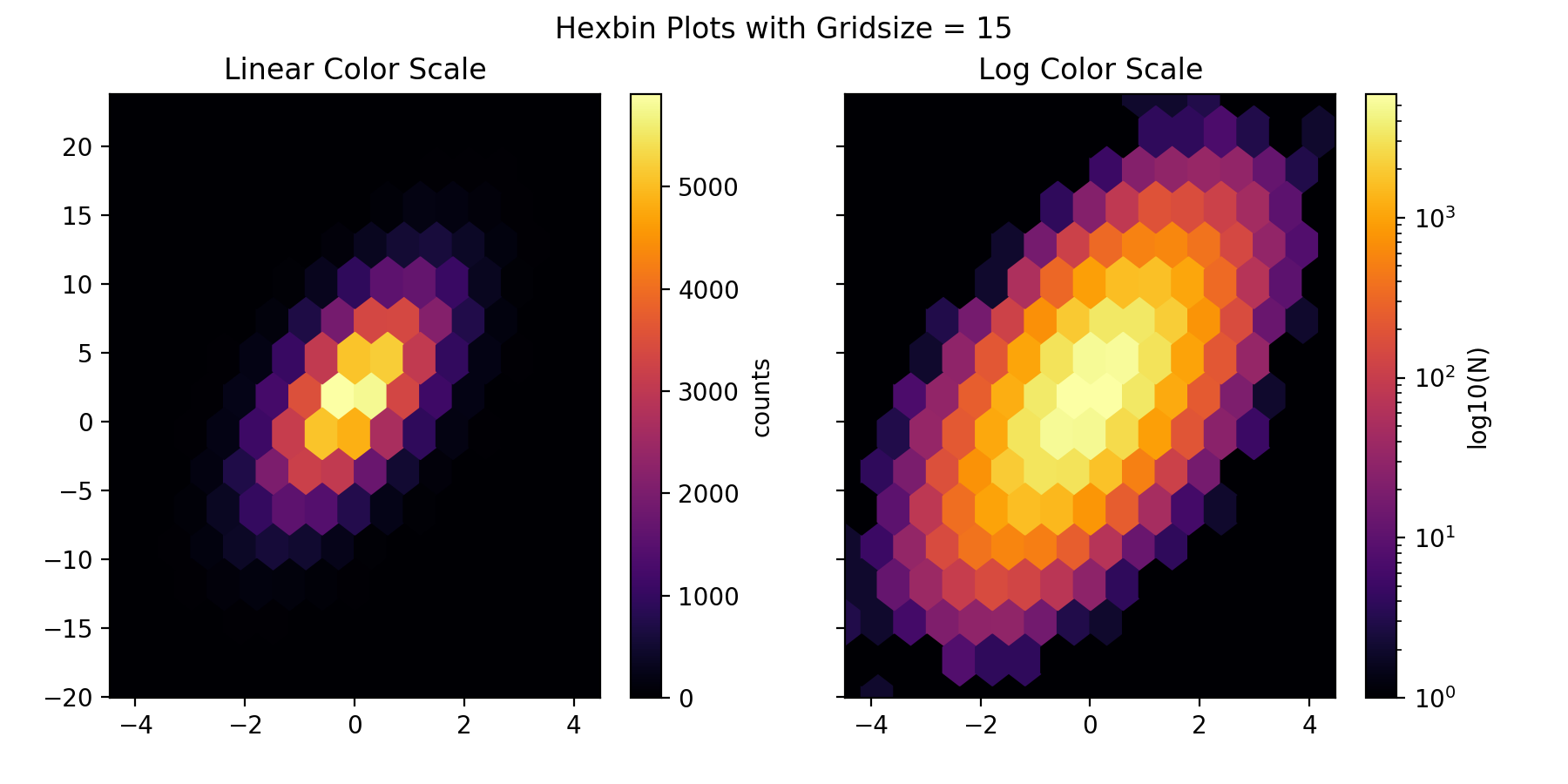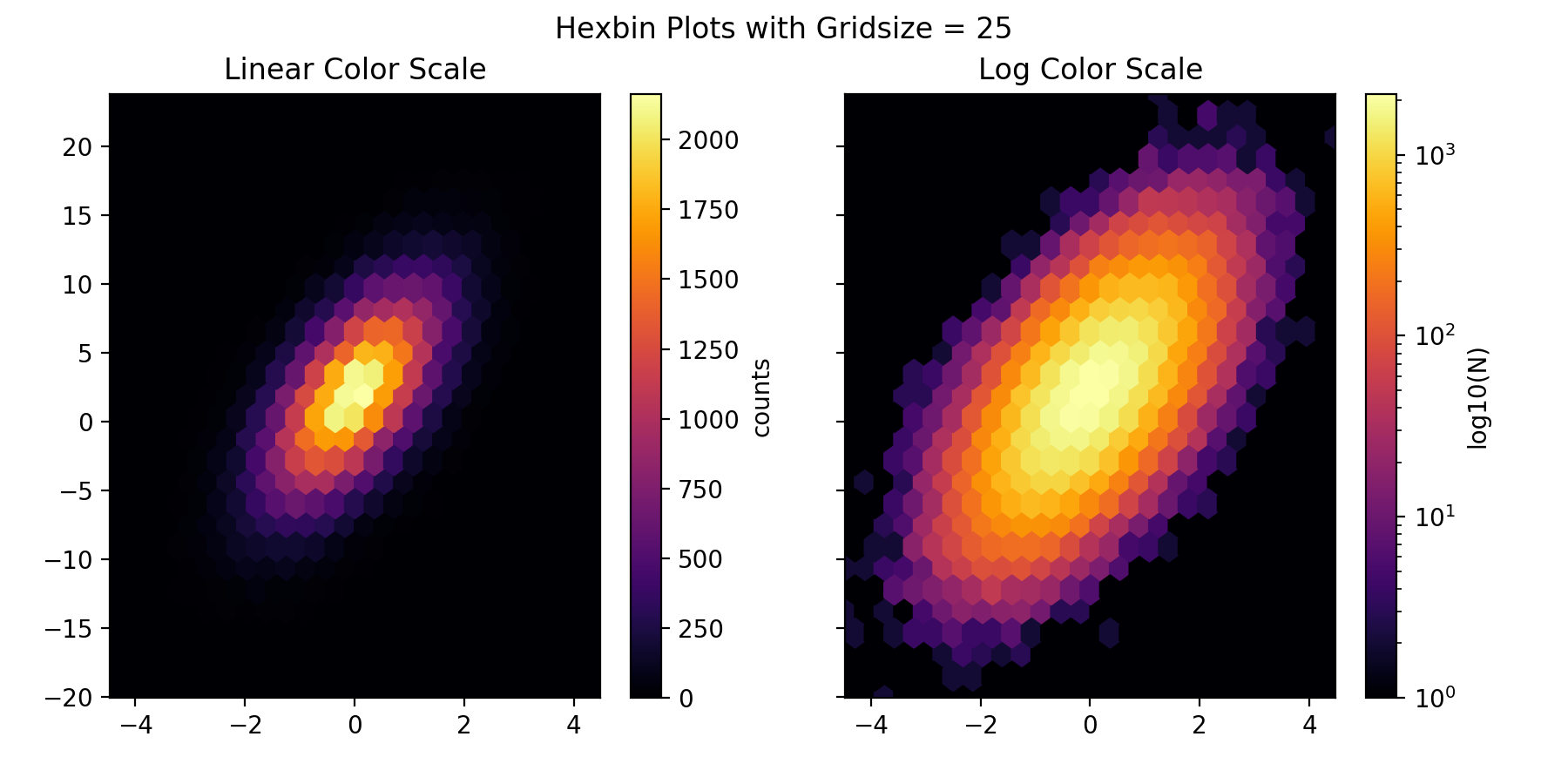# Hexbins

Hexbins are essentially 2-dimensional histograms that leverage color to show the counts (or, “intensity”) of a given region. They can be much more informative than a scatterplot.

Parts of the first example below are taken from a Matplotlib example.

import numpy as np
import matplotlib.pyplot as plt

%matplotlib notebook


### Hexbins versus Scatterplots

Set up data.

# Fixing random state for reproducibility
np.random.seed(42)

n = 100000
x = np.random.standard_normal(n)
y = 2.0 + 3.0 * x + 4.0 * np.random.standard_normal(n)
xmin = x.min()
xmax = x.max()
ymin = y.min()
ymax = y.max()

print(str(x.shape) + ' points are plotted')

100000 points are plotted


### Scatterplots

Note that with this amount of overlap between data points, typical scatterplots are not informative. Even setting the alpha (transparency) so the points are transparent do not show all the possible detail.

fig, axs = plt.subplots(ncols=2,
sharey=True,
figsize=(9, 4))

ax = axs
sc = ax.scatter(x,
y)
ax.axis([xmin, xmax, ymin, ymax])
ax.set_title("Scatterplot without Alpha Set")

ax = axs
alpha=0.01
sc = ax.scatter(x,
y,
alpha=alpha)
ax.axis([xmin, xmax, ymin, ymax])
ax.set_title("Scatterplot with Alpha = {}".format(alpha))

plt.show()

<IPython.core.display.Javascript object>### Hexbins

def plot_hexbin_gridsize(gridsize=50):
fig, axs = plt.subplots(ncols=2,
sharey=True,
figsize=(9, 4.5))
left=0.07,
right=0.93)
ax = axs
hb = ax.hexbin(x, y,
gridsize=gridsize,
cmap='inferno')
ax.axis([xmin, xmax, ymin, ymax])
ax.set_title("Linear Color Scale")
cb = fig.colorbar(hb, ax=ax)
cb.set_label('counts')

ax = axs
hb = ax.hexbin(x, y,
gridsize=gridsize,
bins='log',
cmap='inferno')
ax.axis([xmin, xmax, ymin, ymax])
ax.set_title("Log Color Scale")
cb = fig.colorbar(hb, ax=ax)
cb.set_label('log10(N)')

plt.suptitle('Hexbin Plots with Gridsize = {}'.format(gridsize))
plt.show()

for gridsize in [15, 25, 50, 100]:
plot_hexbin_gridsize(gridsize)

<IPython.core.display.Javascript object><IPython.core.display.Javascript object><IPython.core.display.Javascript object>